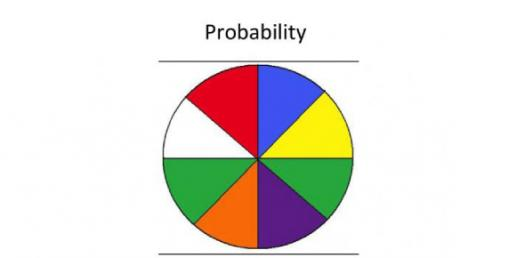Quiz: Can You Pass The Probability Online Test?
15 QuestionsCan you pass the online probability test? An example of probability is to divide the number of events by the number of possible outcomes. This equation will give you the probability of a single event occurring. In the case of rolling a three on a six-sided die, the number of matches is one, and the number of outcomes is six. Then, take this quiz and see what you know.